#2018 iT 邦幫忙鐵人賽DAY 26
1
Data Technology

## 【26】當老闆扎了三下眼問：那可以直接在shiny 上顯示k-means 分類後的地圖嗎?

``````tabsetPanel(
tabPanel("地址表單", tableOutput('addressTable')),
tabPanel("地址分佈圖", plotOutput("allAddressPlot", height = "800px")),
tabPanel("K-means結果分佈圖", plotOutput("classifiedAddressPlot", height = "800px"))
)
``````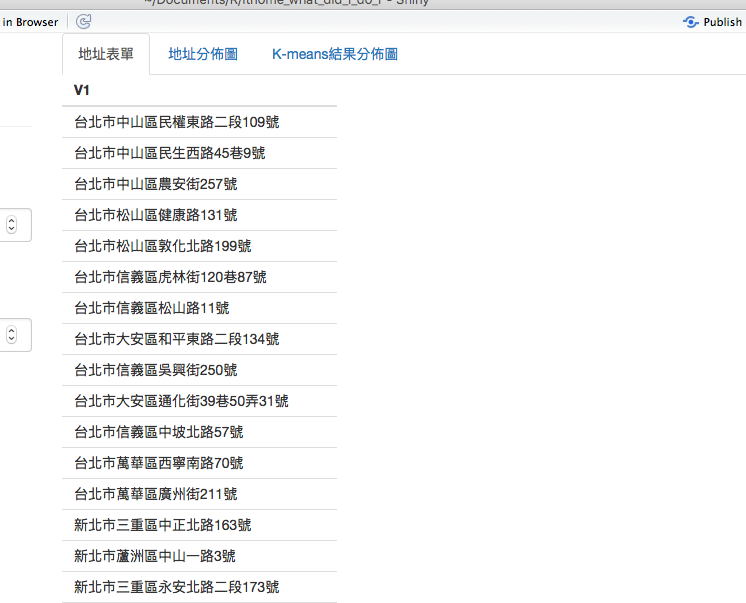``````column(3,
h4("API抓取經緯度"),
actionButton("request", "取資料"),
hr(),
numericInput("seed", h3("種子碼"), value = 20180112),
numericInput("k_value", h3("k值"), value = 10),
actionButton("do", "執行")
)
``````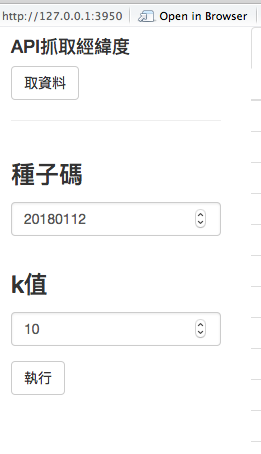``````observeEvent(input\$do, {
if (is.null(v\$addressWithLatLng)) return()

set.seed(input\$seed)
kmeans = kmeans(x = v\$addressWithLatLng[, c('Lat','Lng')], centers = input\$k_value)
v\$addressWithKMeans <- v\$addressWithLatLng %>%
ungroup() %>%
mutate(category=kmeans\$cluster)
})
``````

``````output\$allAddressPlot <- renderPlot({
if (is.null(v\$addressWithLatLng)) return()
ggmap(get_googlemap(center=c(121.52311,25.04126), zoom=12, maptype='satellite'), extent='device') +
geom_point(data=v\$addressWithLatLng, aes(x=Lng, y=Lat), colour='red')
})
``````

``````output\$classifiedAddressPlot <- renderPlot({
if (is.null(v\$addressWithKMeans)) return()
ggmap(get_googlemap(center=c(121.52311,25.04126), zoom=12, maptype='satellite'), extent='device') +
geom_point(data=v\$addressWithKMeans,
size=1.8,
aes(x=Lng, y=Lat, colour=factor(category)))
})
``````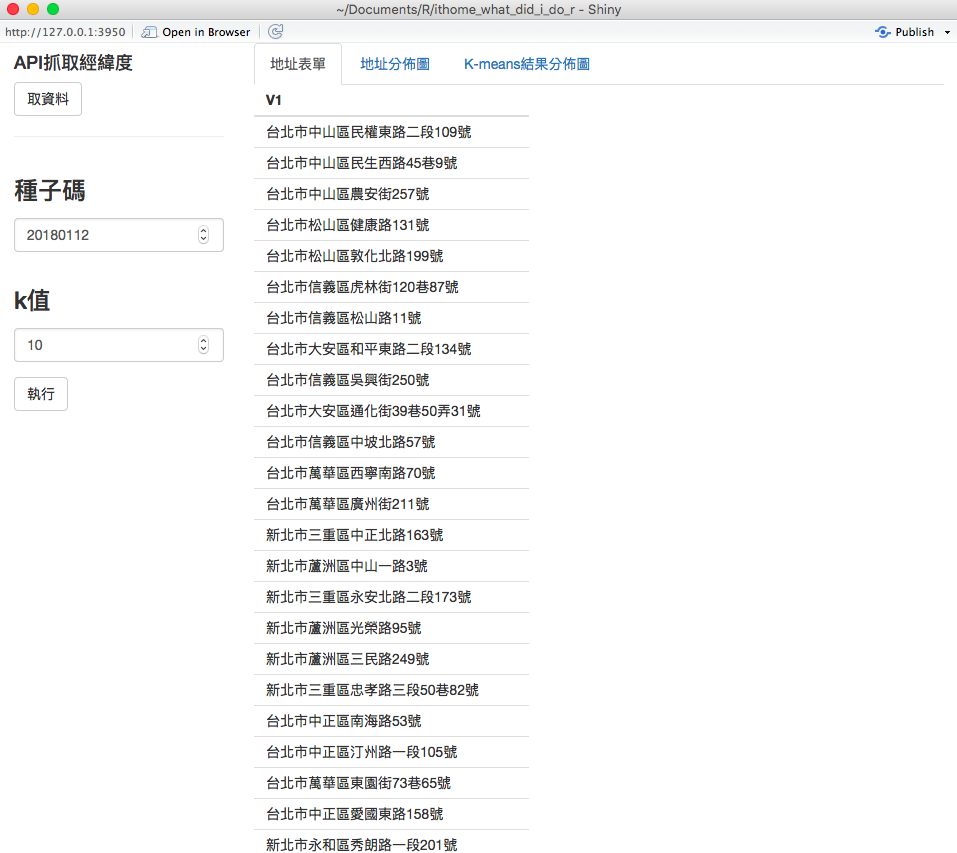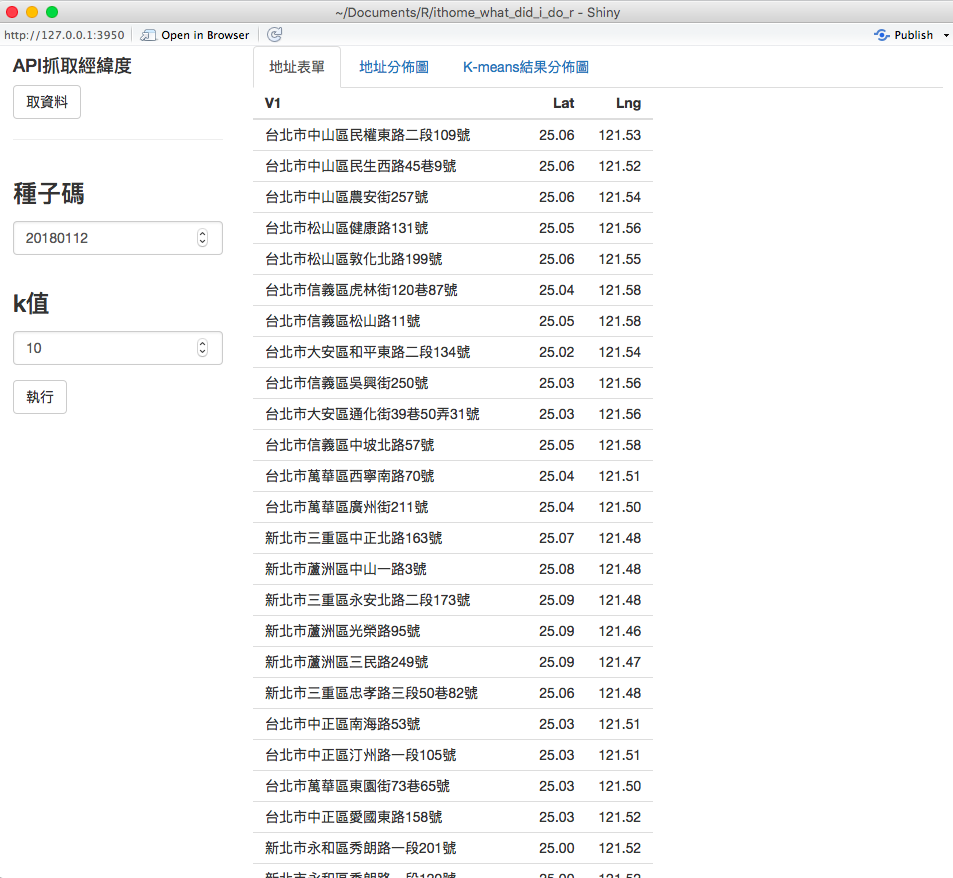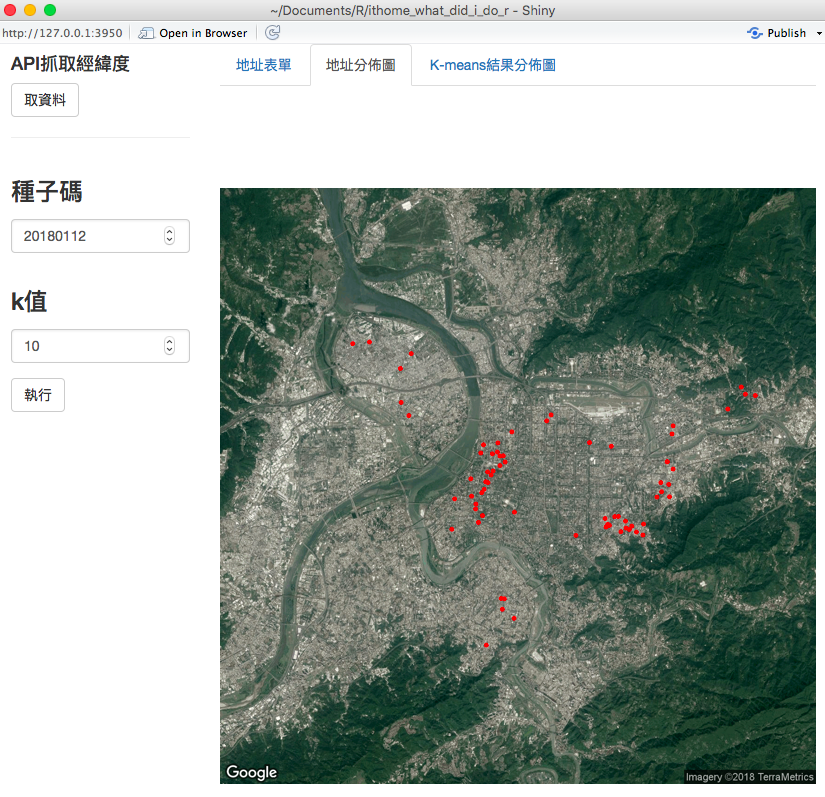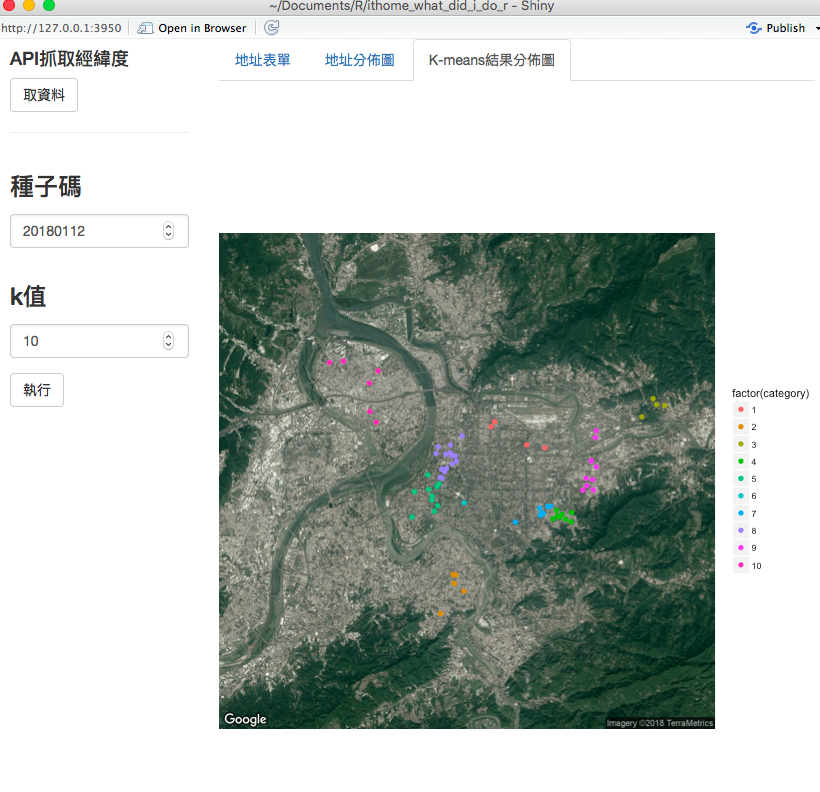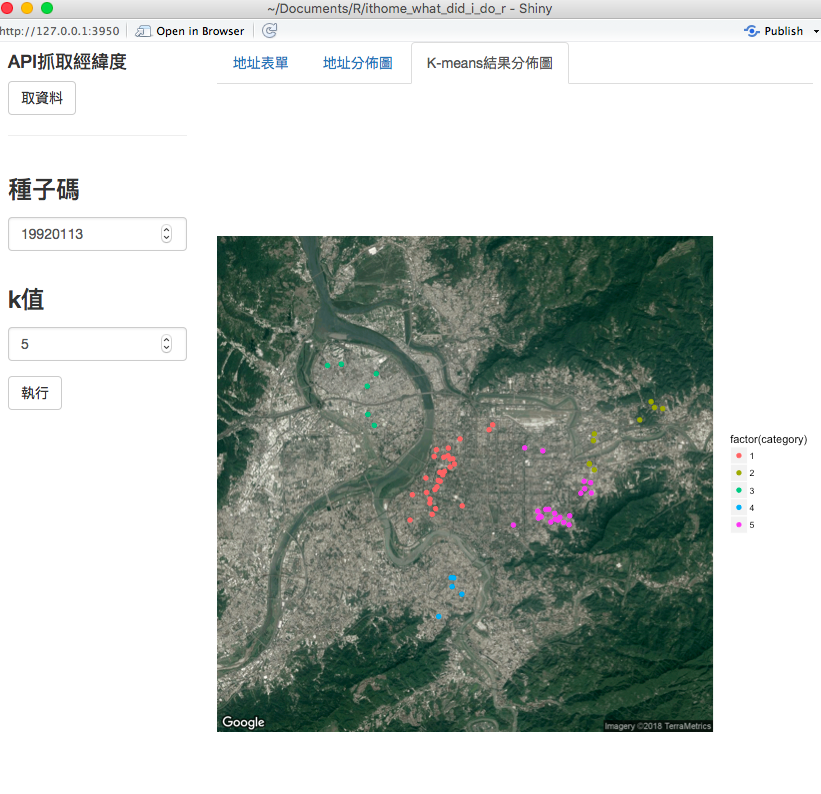ref:
day26原始碼